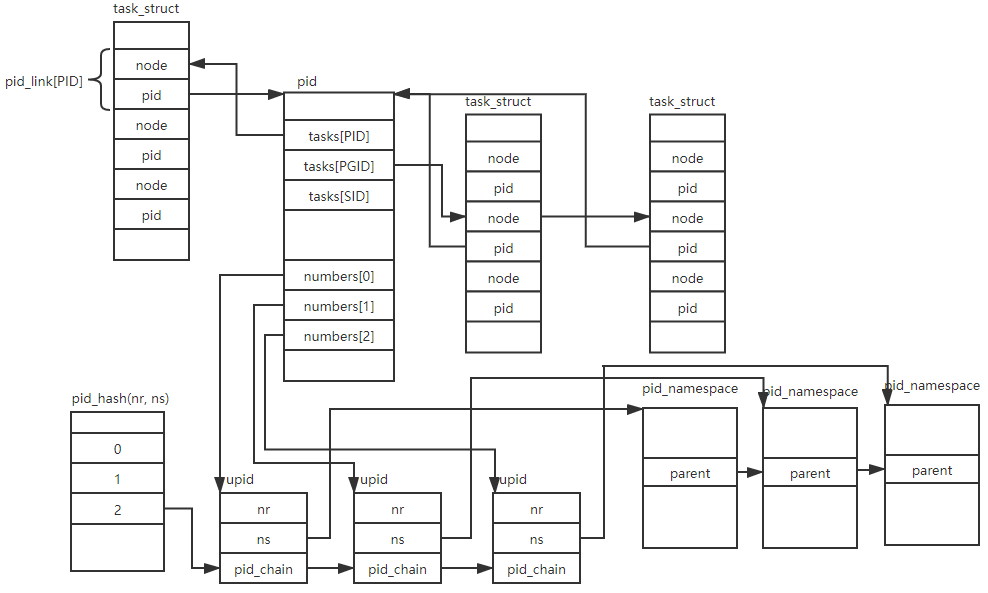2019-12-20

Namespace is another method to abstract resouces. A namespace make it appear to the process within the namespace that they have their own isolated instance of the global resouces. Compared to the virtual machine, namespace is more lightweight. In this post, I will dig into the pid namespace from kernel part. I used kernel 4.4 in this post.

### Basic structure

There are six different types of namespaces, they are uts, ipc, mount, pid, net and user. pid namespace is structured together in ‘nsproxy’ structure.

``````struct nsproxy {
atomic_t count;
struct uts_namespace *uts_ns;
struct ipc_namespace *ipc_ns;
struct mnt_namespace *mnt_ns;
struct pid_namespace *pid_ns_for_children;
struct net 	     *net_ns;
};
``````

‘task_struct’ has a ‘nsproxy’ member pointing to a ‘struct nsproxy’ to represent the process’s resource view. struct ‘pid_namespace’ is used to represent a pid namespace.

``````    struct pid_namespace {
struct kref kref;
struct pidmap pidmap[PIDMAP_ENTRIES];
int last_pid;
unsigned int nr_hashed;
struct kmem_cache *pid_cachep;
unsigned int level;
struct pid_namespace *parent;
#ifdef CONFIG_PROC_FS
struct vfsmount *proc_mnt;
struct dentry *proc_self;
#endif
#ifdef CONFIG_BSD_PROCESS_ACCT
struct fs_pin *bacct;
#endif
struct user_namespace *user_ns;
struct work_struct proc_work;
kgid_t pid_gid;
int hide_pid;
int reboot;	/* group exit code if this pidns was rebooted */
struct ns_common ns;
};
``````

pidmap member is a struct ‘pidmap’, it’s a bitmap to be used to managing pid value. It’s definition is quite easy.

``````    struct pidmap {
atomic_t nr_free;
void *page;
};
``````

‘last_pid’ record the last used pid value. ‘child_reper’ is the init process of a pid_namespace. ‘user_ns’ points the user namespace of this pid namespace.

pid namespace is created by function ‘create_pid_namespace’ in the call chain of clone->copy_namespaces->copy_pid_ns->create_pid_namespace.

``````    static struct pid_namespace *create_pid_namespace(struct user_namespace *user_ns,
struct pid_namespace *parent_pid_ns)
{
struct pid_namespace *ns;
unsigned int level = parent_pid_ns->level + 1;
int i;
int err;

if (level > MAX_PID_NS_LEVEL) {
err = -EINVAL;
goto out;
}

err = -ENOMEM;
ns = kmem_cache_zalloc(pid_ns_cachep, GFP_KERNEL);
if (ns == NULL)
goto out;

ns->pidmap.page = kzalloc(PAGE_SIZE, GFP_KERNEL);
if (!ns->pidmap.page)
goto out_free;

ns->pid_cachep = create_pid_cachep(level + 1);
if (ns->pid_cachep == NULL)
goto out_free_map;

err = ns_alloc_inum(&ns->ns);
if (err)
goto out_free_map;
ns->ns.ops = &pidns_operations;

kref_init(&ns->kref);
ns->level = level;
ns->parent = get_pid_ns(parent_pid_ns);
ns->user_ns = get_user_ns(user_ns);
INIT_WORK(&ns->proc_work, proc_cleanup_work);

set_bit(0, ns->pidmap.page);
atomic_set(&ns->pidmap.nr_free, BITS_PER_PAGE - 1);

for (i = 1; i < PIDMAP_ENTRIES; i++)
atomic_set(&ns->pidmap[i].nr_free, BITS_PER_PAGE);

return ns;
}
``````

This function is quite easy, ‘ns->pidmap.page’ is the bitmap used to allocate/delete pid value.

‘create_pid_cachep’ is used to create the ‘struct pid’ cache. It is defined as following:

``````    struct pid
{
atomic_t count;
unsigned int level;
/* lists of tasks that use this pid */
struct upid numbers;
};

struct upid {
/* Try to keep pid_chain in the same cacheline as nr for find_vpid */
int nr;
struct pid_namespace *ns;
struct hlist_node pid_chain;
};
``````

Every process has a ‘struct pid’,

Following pic shows the data structure relation. A process may reference another ‘pid’, the ‘task’s in ‘struct pid’ is used for this. ‘struct upid’ stores the pid value in ‘nr’ member, the ‘pid_chain’ member is used to link the ‘struct upid’ in ‘pid_hash’ hash table.

For a process, it has a ‘level+1’ pid value, one in his pid namespace, and one for every his parent pid namespace. So in ‘create_pid_cachep’, it allocates a ‘struct pid’ and ‘level’ numbers of ‘struct upid’.

``````    static struct kmem_cache *create_pid_cachep(int nr_ids)
{
struct pid_cache *pcache;
struct kmem_cache *cachep;

mutex_lock(&pid_caches_mutex);
list_for_each_entry(pcache, &pid_caches_lh, list)
if (pcache->nr_ids == nr_ids)
goto out;

pcache = kmalloc(sizeof(struct pid_cache), GFP_KERNEL);
if (pcache == NULL)
goto err_alloc;

snprintf(pcache->name, sizeof(pcache->name), "pid_%d", nr_ids);
cachep = kmem_cache_create(pcache->name,
sizeof(struct pid) + (nr_ids - 1) * sizeof(struct upid),
0, SLAB_HWCACHE_ALIGN, NULL);
if (cachep == NULL)
goto err_cachep;

pcache->nr_ids = nr_ids;
pcache->cachep = cachep;
out:
mutex_unlock(&pid_caches_mutex);
return pcache->cachep;
}
``````

### pid management

‘struct pid’ is created by ‘alloc_pid’ called by ‘copy_process’.

``````    struct pid *alloc_pid(struct pid_namespace *ns)
{
struct pid *pid;
enum pid_type type;
int i, nr;
struct pid_namespace *tmp;
struct upid *upid;
int retval = -ENOMEM;

pid = kmem_cache_alloc(ns->pid_cachep, GFP_KERNEL);
tmp = ns;
pid->level = ns->level;
for (i = ns->level; i >= 0; i--) {
nr = alloc_pidmap(tmp);
if (IS_ERR_VALUE(nr)) {
retval = nr;
goto out_free;
}

pid->numbers[i].nr = nr;
pid->numbers[i].ns = tmp;
tmp = tmp->parent;
}

if (unlikely(is_child_reaper(pid))) {
if (pid_ns_prepare_proc(ns)) {
disable_pid_allocation(ns);
goto out_free;
}
}

get_pid_ns(ns);
atomic_set(&pid->count, 1);
for (type = 0; type < PIDTYPE_MAX; ++type)

upid = pid->numbers + ns->level;
spin_lock_irq(&pidmap_lock);
goto out_unlock;
for ( ; upid >= pid->numbers; --upid) {
&pid_hash[pid_hashfn(upid->nr, upid->ns)]);
upid->ns->nr_hashed++;
}
spin_unlock_irq(&pidmap_lock);

return pid;
}
``````

Every process has ‘level+1’ pid value, one for every namespace that can see this process. In the first for loop, ‘alloc_pidmap’ return the pid value for this process in pid_namespace ‘tmp’. In the last for loop, we use the ‘upid->nr’ and ‘upid->ns’ as the key and insert the ‘struct upid’ to the crosspending ‘pid_hash’ table. In this function, we also initialize ‘pid->tasks’ list head. This list head is used to link the process that uses this ‘struct pid’. ‘struct pid_link’ is used to link the ‘task_struct’ and ‘pid’.

``````    struct pid_link
{
struct hlist_node node;
struct pid *pid;
};
``````

Here ‘node’ is the list entry links to ‘pid->tasks’. And ‘pid’ point to the ‘struct pid’. In ‘copy_process’, we can see following code:

``````    if (likely(p->pid)) {
ptrace_init_task(p, (clone_flags & CLONE_PTRACE) || trace);

if (is_child_reaper(pid)) {
ns_of_pid(pid)->child_reaper = p;
p->signal->flags |= SIGNAL_UNKILLABLE;
}

p->signal->tty = tty_kref_get(current->signal->tty);
attach_pid(p, PIDTYPE_PGID);
attach_pid(p, PIDTYPE_SID);
__this_cpu_inc(process_counts);
} else {
atomic_inc(&current->signal->live);
atomic_inc(&current->signal->sigcnt);
}
attach_pid(p, PIDTYPE_PID);
}

static inline void
{
}

{
}

{